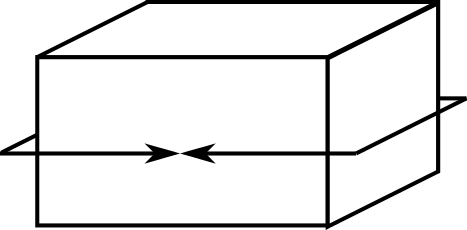#### You may also like### 2-digit Square

A 2-Digit number is squared. When this 2-digit number is reversed and squared, the difference between the squares is also a square. What is the 2-digit number?### Consecutive Squares

The squares of any 8 consecutive numbers can be arranged into two sets of four numbers with the same sum. True of false?### Plus Minus

Can you explain the surprising results Jo found when she calculated the difference between square numbers?

# Cuboid Perimeters

##### Age 14 to 16 Short Challenge Level:

The 'perimeter' of a cuboid can be measured along three axes.

One 'perimeter' of this cuboid is shown.

If the 'perimeters' of a cuboid are 12 cm, 16 cm and 24 cm, what is the volume of the cuboid?

This problem is adapted from the World Mathematics Championships
You can find more short problems, arranged by curriculum topic, in our short problems collection.